# 10.P: Problems

$$\newcommand{\vecs}{\overset { \rightharpoonup} {\mathbf{#1}} }$$ $$\newcommand{\vecd}{\overset{-\!-\!\rightharpoonup}{\vphantom{a}\smash {#1}}}$$$$\newcommand{\id}{\mathrm{id}}$$ $$\newcommand{\Span}{\mathrm{span}}$$ $$\newcommand{\kernel}{\mathrm{null}\,}$$ $$\newcommand{\range}{\mathrm{range}\,}$$ $$\newcommand{\RealPart}{\mathrm{Re}}$$ $$\newcommand{\ImaginaryPart}{\mathrm{Im}}$$ $$\newcommand{\Argument}{\mathrm{Arg}}$$ $$\newcommand{\norm}{\| #1 \|}$$ $$\newcommand{\inner}{\langle #1, #2 \rangle}$$ $$\newcommand{\Span}{\mathrm{span}}$$ $$\newcommand{\id}{\mathrm{id}}$$ $$\newcommand{\Span}{\mathrm{span}}$$ $$\newcommand{\kernel}{\mathrm{null}\,}$$ $$\newcommand{\range}{\mathrm{range}\,}$$ $$\newcommand{\RealPart}{\mathrm{Re}}$$ $$\newcommand{\ImaginaryPart}{\mathrm{Im}}$$ $$\newcommand{\Argument}{\mathrm{Arg}}$$ $$\newcommand{\norm}{\| #1 \|}$$ $$\newcommand{\inner}{\langle #1, #2 \rangle}$$ $$\newcommand{\Span}{\mathrm{span}}$$$$\newcommand{\AA}{\unicode[.8,0]{x212B}}$$

### Q19.2.1

Indicate the coordination number for the central metal atom in each of the following coordination compounds:

1. [Pt(H2O)2Br2]
2. [Pt(NH3)(py)(Cl)(Br)] (py = pyridine, C5H5N)
3. [Zn(NH3)2Cl2]
4. [Zn(NH3)(py)(Cl)(Br)]
5. [Ni(H2O)4Cl2]
6. [Fe(en)2(CN)2]+ (en = ethylenediamine, C2H8N2)

### S19.2.1

First we must identify whether or not the ligand has more than one bonded atom (bidentate/polydentate). Using the table below we are able to do this.

Ligand Number of bonded atoms
Ammine (NH3) monodentate
Aqua (H2O) monodentate
Bromo (Br) monodentate
Chloro (Cl) monodentate
Cyano (CN) monodentate
Pyridine (C5H5N) monodentate
Ethylenediamine (C2H8N2) bidentate

Now that we have identified the number of bonded atoms from each ligand, we can find the total amount of bonded atoms to the central metal ion, giving us the coordination number.

1. $$[Pt(H_2O)_2Br_2]$$: We can identify the metal ion in the complex at Pt, platinum, as the other two are listed as ligands above and are nonmetallic. We can now use the number of ligands and their bonding atoms to find its coordination number. From the table above we see that H2O has only one bonding atom and Br as well. So for each Br atom we have one bonding atom, and we have two of these, yielding 2 bonding atoms, this is the same for H2O, giving us a total number of 4 bonding atoms, and therefore a coordination number of 4.
2. $$[Pt(NH_3)(py)(Cl)(Br)]$$ (py = pyridine, C5H5N): The metal ion in this complex, similarly to the first one, can be identified as Pt, platinum. The ligands can be identified as NH3, pyridine, Cl, and Br, which are all monodentate ligands and have one bonding atom each. Since we have four ligands, each with one bonding atom, the total number of bonding atoms on the metal ion is 4, therefore the complex has a coordination number of 4.
3. $$[Zn(NH_3)_2Cl_2]$$: The metal ion in this complex can be identified as Zn, zinc, and the ligands can be identified as NH3 and Cl. Since these two are both monodentate ligands they have one bonding atom each. Since we have a total of two NH3 and two Cl ligands, we get a total of four monodentate ligands, giving us 4 bonding atoms and a coordination number of 4.
4. $$[Zn(NH_3)(py)(Cl)(Br)]$$: The metal ion in this complex can be identified as Zn, zinc, and the ligands can be identified as NH3, pyridine, Cl, and Br, which are all monodentate ligands and have one bonding atom each. Since we have four ligands, each with one bonding atom, the total number of bonding atoms on the metal ion is 4, therefore the complex has a coordination number of 4.
5. $$[Ni(H_2O)_4Cl_2]$$: The metal ion in this complex can be identified as Ni, nickel, and we can now use the number of ligands and their bonding atoms to find its coordination number. From the table above we see that H2O has only one bonding atom and Cl as well. So for each Cl atom we have one bonding atom, and we have two of these, yielding 2 bonding atoms. H2O is the same, having only one bonding atom, but there are four of these. So this gives us a total number of 6 bonding atoms, and therefore a coordination number of 6.
6. $$[Fe(en)_2(CN)_2]^+$$ (en = ethylenediamine, C2H8N2): The metal ion in this complex can be identified as Fe, iron, and the ligands can be identifies as (en) and CN. Since (en) is bidentate, meaning it has 2 bonding atoms, and there are two of these the total bonding atoms from (en) is four. Since CN is monodentate, meaning it has one bonding atom, and there are two of these, the total bonding atoms from CN ligand is two. So, the total amount of bonding atoms is 6, therefore the complex has a coordination number of 6.

### A19.2.1

1. The 2 aqua and the 2 bromo ligands form a total of 4 coordinate covalent bonds and as a result the coordination number is 4.
2. The ammine, pyridine, chloro and bromo each form one coordinate covalent bond that gives a total of 4 and hence CN=4
3. Two ammine and two chloro ligands give a total of 4 coordinate covalent bonds and a CN = 4.
4. One ammine, a pyrimidine, a chloro and a bromo ligand give a total of 4 covalent bonds, resulting in CN = 4.
5. Four aqua ligands and two chloro ligands form a total of 6 coordinate covalent bonds and a CN =6
6. Ethylenediamine is a bidentate ligand that forms two coordinate covalent bonds and along with two cyano ligands it forma a total of 6 bonds. hence has a CN=6

### Q19.2.2

Give the coordination numbers and write the formulas for each of the following, including all isomers where appropriate:

1. tetrahydroxozincate(II) ion (tetrahedral)
3. dichloroaurate ion (note that aurum is Latin for "gold")
4. diaminedichloroplatinum(II)
5. potassium diaminetetrachlorochromate(III)
6. hexaaminecobalt(III) hexacyanochromate(III)
7. dibromobis(ethylenediamine) cobalt(III) nitrate

### S19.2.2

To determine coordination numbers we must count the total number of ligands bonded to the central metal and distinguish monodentate and polydentate ligands. To determine the formulas, we use the nomenclature rules and work backwards

1. "tetrahydroxo" = 4 hydroxide ligands, since hydroxide is a monodentate ligand, we have a total of 4 bonds to the central metal
Coordination Number: ​​​​​​4
We review the basics of nomenclature and see that "tetra" = 4 and "hydroxo" = OH-. Since the charge on Zinc is 2+ which is given in the nomenclature by the roman numerals, we can calculate the total charge on the complex is 2-
Formula: [Zn(OH)4]2−
2. "hexacyano" = 6 cyanide ligands, since cyanide is a monodentate ligand, we have a total of 6 bonds to the central metal
Coordination Number: 6
We review the basics of nomenclature and see that "hexa" = 6 and "cyano" = CN-. Since the charge on Pd is 4+ which is given in the nomenclature by the roman numerals, we can calculate the total charge on the complex is 2-
Formula: [Pd(CN)6]2−
3. "dicholor" = 2 chloride ligands, since chloride is a monodentate ligand, we have a total of 2 bonds to the central metal
Coordination Number: 2
We review the basics of nomenclature and see that "di" = 2 and "chloro" = Cl-. Since the charge on Au is always 1+, we can calculate the total charge on the complex is 1-
Formula: [AuCl2]
4. "diamine" = 2 ammonia ligands and "dichloro" = 2 chloride ligands, since both ammonia and chloride ligands are monodentant, we have a total of 4 bonds to the central metal
Coordination Number: 4
We review the basics of nomenclature and see that "di" = 2, "chloro" = Cl- and "amine" = NH3. Since the charge on Pt is 2+ which is given in the nomenclature by the roman numerals, we can calculate that the total charge is 0 so the complex is neutral.
Formula: [Pt(NH3)2Cl2]
5. "diamine" = 2 ammonia ligands and "tetrachloro" = 4 chloride ligands since both ammonia and chloride ligands are monodentant, we have a total of 6 bonds to the central metal
Coordination Number: 6
We review the basics of nomenclature and see that "di" = 2, "amine" = NH3, "tetra" = 4 and "chloro" = Cl-. Since the charge on the central metal, Cr, is 3+ which is given in the nomenclature by the roman numerals, we can calculate that the total charge of the complex is 1-. The "potassium" at the front of the nomenclature indicates that it is the corresponding cation to this anionic complex.
Formula: K[Cr(NH3)2Cl4]
6. Both of the metal complexes have "hexa" monodentate ligands which means both have coordination numbers of 6.
Coordination Number: 6
We review the basics of nomenclature and see that "hexa" = 6, "amine" = NH3, and "cyano" = CN-. Since the charge on Cr is 3+ and Co is 3+ which is given in the nomenclature by the roman numerals, we find that these complexes charges balance out.
Formula: [Co(NH3)6][Cr(CN)6]
7. "dibromo" = 2 bromide ligands "bis(ethylenediamine)" = 2 (en) ligands; Bromide is a monodentate ligand while (en) is a bidentate ligand. Therefore; we have a coordination number of 6.
Coordination Number: 6
We review the basics of nomenclature and see that "di" = 2, "bromo" = Br-, "bis" = 2, and "ethyllenediamine" = en. Since the charge on Co is 3+ which is given in the nomenclature by the roman numerals. Nitrate is the corresponding anion to this cationic complex.
Formula: [Co(en)2Br2]NO3

### A19.2.2

1. 4, [Zn(OH)4]2−;
2. 6, [Pd(CN)6]2−;
3. 2, [AuCl2];
4. 4, [Pt(NH3)2Cl2];
5. 6, K[Cr(NH3)2Cl4];
6. 6, [Co(NH3)6][Cr(CN)6];
7. 6, [Co(en)2Br2]NO3

### Q19.2.3

Give the coordination number for each metal ion in the following compounds:

1. [Co(CO3)3]3− (note that CO32− is bidentate in this complex)
2. [Cu(NH3)4]2+
3. [Co(NH3)4Br2]2(SO4)3
4. [Pt(NH3)4][PtCl4]
5. [Cr(en)3](NO3)3
6. [Pd(NH3)2Br2] (square planar)
7. K3[Cu(Cl)5]
8. [Zn(NH3)2Cl2]

### S19.2.3

You can determine a compound's coordination number based on how many ligands are bound to the central atom.

1) In this compound, Cobalt is the central atom, and it has 3 CO32- molecules attached to it. However, CO32- is a bidentate ligand, which means it binds to the central atom in two places rather than one. This means that the coordination number of [Co(CO3)3]3- is 6. A coordination number of 6 means that the structure is most likely octahedral.

2) In this compound, Copper is the central atom. 4 ammonia molecules are attached to it. This means the coordination number is 4, and the structure is likely tetrahedral.

3) For this compound, we can ignore the (SO4)3 because it is not bound to the central atom. The central atom is cobalt, and it has 4 ammonia molecules and 2 bromine molecules bound to it. The coordination number is 6.

4) There are two compounds here, indicated by the brackets. The central atom for both is platinum. One of them has 4 ammonia molecules attached, and the other has 4 chlorine atoms attached. Both complexes have a coordination number of 4.

5) We can ignore (NO3)3 for this compound. The central atom is Chromium. There are 3 ethylenediamine molecules attached to the chromium. Ethylenediamine is a bidentate ligand, so the coordination number is 6.

6) Palladium is the central atom. 2 ammonia molecules and 2 bromine atoms are bound to the palladium atom. The coordination number is 4.

7) We can ignore the K3 structure. Copper is the central atom, and there are 5 chlorine molecules attached to it. The coordination number is 5, so the structure is either trigonal bipyramidal or square pyramidal.

8) In this compound, zinc is the central atom. There are 2 ammonia molecules and 2 chlorine atoms attached. This means that the coordination number is 4.

Q19.2.4

Sketch the structures of the following complexes. Indicate any cis, trans, and optical isomers.

1. [Pt(H2O)2Br2] (square planar)
2. [Pt(NH3)(py)(Cl)(Br)] (square planar, py = pyridine, C5H5N)
3. [Zn(NH3)3Cl]+ (tetrahedral)
4. [Pt(NH3)3Cl]+ (square planar)
5. [Ni(H2O)4Cl2]
6. [Co(C2O4)2Cl2]3− (note that $$\ce{C2O4^2-}$$ is the bidentate oxalate ion, $$\ce{−O2CCO2-}$$)

### S19.2.4

Cis and trans are a type of geometric isomer, meaning there is a difference in the orientation in which the ligands are attached to the central metal. In cis, two of the same ligands are adjacent to one another and in trans, two of the same ligands are directly across from one another.

Optical isomers → have the ability to rotate light, optical isomers are also chiral. Only chiral complexes have optical isomers

Chiral → asymmetric, structure of its mirror image is not superimposable

Enantiomers: chiral optical isomers (compound can have multiple enantiomers)

Tetrahedral complex with 4 distinct ligands → always chiral

• For tetrahedral, if 2 ligands are the same, then it cannot be chiral, has a plane of symmetry

Solutions:

a. $$[Pt(H_2O)_2Br_2]$$ (square planar)

This complex has 2 kinds of ligands. The matching ligands can either be adjacent to each other and be cis, or they can be across from each other and be trans.

b. $$[Pt(NH_3)(py)(Cl)(Br)]$$ (square planar, py = pyridine, $$C_5H_5N$$)

This complex has 4 different ligands. There is no plane of symmetry in any of the enantiomers, making the structures chiral and therefore has optical isomers.

c. $$[Zn(NH_3)_3Cl]^+$$ (tetrahedral)

There is a plane of symmetry from $$NH_3$$ through $$Zn$$ to the other $$NH_3$$, therefore it is not chiral.

d. $$[Pt(NH_3)_3Cl]^+$$ (square planar)

There is a plane of symmetry from $$NH_3$$ through $$Pt$$ to the other $$NH_3$$, therefore it is not chiral.

e. $$[Ni(H_2O)_2Cl_2]$$

The $$Cl$$ ligands can either be right next to each other, or directly across from one another allowing for both cis and trans geometries.

f. $$[Co(C_2O_4)_2Cl_2]^-3$$ (note that $$C_2O_4^-2$$ is the bidentate oxalate ion, $$^−O_2CCO_2^-$$

There is a plane of symmetry from $$Cl$$ through Co to the other $$Cl$$ in a "trans" chlorine configuration, therefore it is not chiral in a chlorine "trans" configuration. However, there is no symmetry in the chlorine "cis" configuration, indicating multiple "cis" isomers.

### A19.2.4

a. [Pt(H2O)2Br2]:b. [Pt(NH3)(py)(Cl)(Br)]: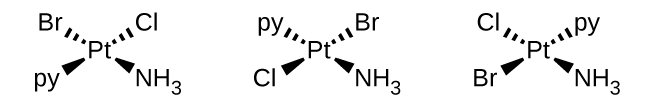c. [Zn(NH3)3Cl]+ :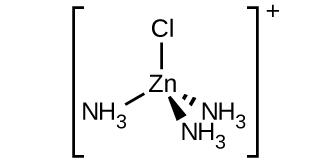d. [Pt(NH3)3Cl]+ :e. [Ni(H2O)4Cl2]: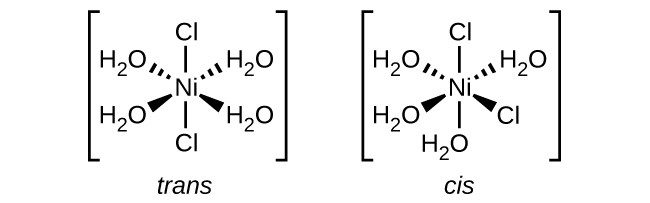f. [Co(C2O4)2Cl2]3−:### Q19.2.5

Draw diagrams for any cis, trans, and optical isomers that could exist for the following (en is ethylenediamine):

1. [Co(en)2(NO2)Cl]+
2. [Co(en)2Cl2]+
3. [Pt(NH3)2Cl4]
4. [Cr(en)3]3+
5. [Pt(NH3)2Cl2]

### S19.2.5

We are instructed to draw all geometric isomers and optical isomers for the specified compound. Optical isomers exist when an isomer configuration is not superimposable on its mirror image. This means there are two distinct molecular shapes. Often a left and right hand are cited as an example; if you were to take your right hand and place it upon your left, you cannot make the major parts of your hand align on top of one another. The basic idea when deciding whether something is optically active is to look for a plane of symmetry--if you are able to bisect a compound in a manner that establishes symmetry, then the compound does not have an optical isomer.

Cis isomers exist when there are 2 ligands of the same species placed at 90 degree angles from each other. Trans isomers exist when there are 2 ligands of the same species placed at 180 degree angles from each other.

Problem 1

This compound is an octahedral molecule, so the six ligands (atoms in the complex that are not the central transition metal) are placed around the central atom at 90 degree angles. Two optical isomers exist for [Co(en)2(NO2)Cl]+. The second isomer is drawn by taking the mirror image of the first.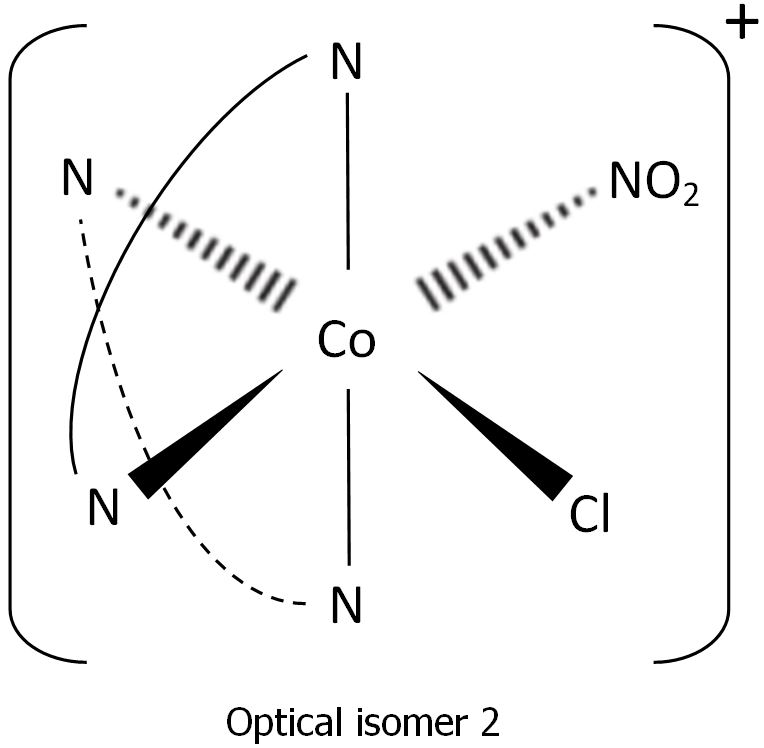Problem 2

This compound is also an octahedral molecule. Two cis (optical) isomers and one trans isomer exist for [Co(en)2Cl2]+. The trans isomer can be drawn by placing the chlorine ligands in positions where they form a 180 degree angle with the central atom. The first cis isomer can be drawn by placing the chlorine ligands in positions where they form a 90 degree angle with the central atom. The second cis isomer can be found by mirroring the first cis isomer, like we did in problem 1.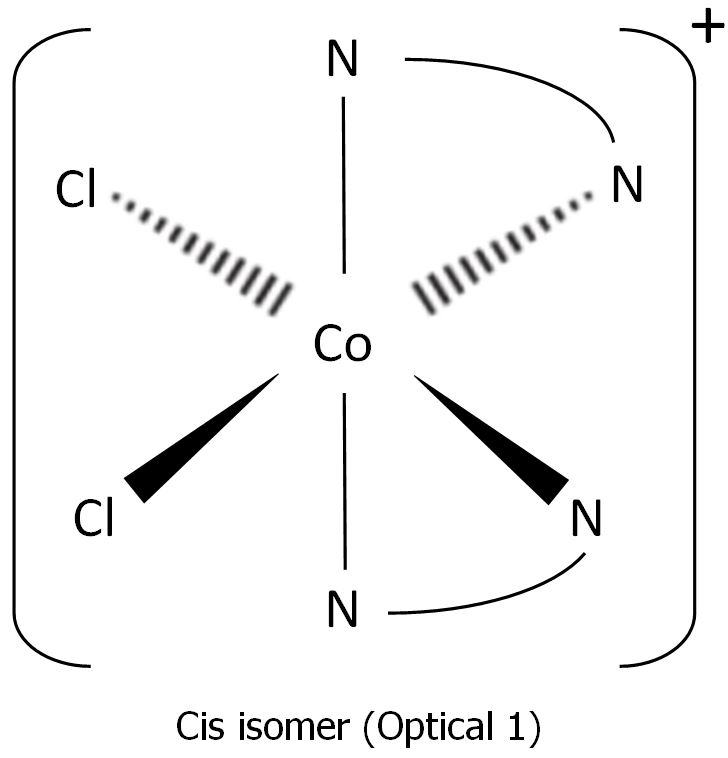Problem 3

This compound is also an octahedral molecule. One trans isomer and one cis isomer of [Pt(NH3)2Cl4] exist. The trans isomer can be drawn by placing the ammonia ligands in positions where they form a 180 degree angle with the central atom. The cis isomer can be drawn by placing the ammonia ligands in positions where they form a 90 degree angle with the central atom.Problem 4

This compound is also an octahedral molecule. Two optical isomers for [Cr(en)3]3+ exist. The second optical isomer can be drawn by taking the mirror image of the first optical isomer.Problem 5

This compound is a square planar complex, so the ligands are placed around the central atom in a plane, at 90 angles. A trans isomer and a cis isomer exist for the complex [Pt(NH3)2Cl2]. The trans isomer can be drawn by placing the ammonia ligands in positions where they form a 180 degree angle in the plane with the central atom. The cis isomer can be drawn by placing the ammonia ligands in positions where they form a 90 degree angle in the plane with the central atom.### Q19.2.6

Name each of the compounds or ions given in Exercise Q19.2.3, including the oxidation state of the metal.

### S19.2.6

Rules to follow for coordination complexes

1. Cations are always named before the anions.

2. Ligands are named before the metal atom or ion.

3. Ligand names are modified with an ‐o added to the root name of an anion. For neutral ligands the name of the molecule is used, with the exception of OH2, NH3, CO and NO.

4. The prefixes mono‐, di‐, tri‐, tetra‐, penta‐, and hexa‐ are used to denote the number of simple ligands.

5. The prefixes bis‐, tris‐, tetrakis‐, etc., are used for more complicated ligands or ones that already contain di‐, tri‐, etc.

6. The oxidation state of the central metal ion is designated by a Roman numeral in parentheses.

7. When more than one type of ligand is present, they are named alphabetically. Prefixes do not affect the order.

8. If the complex ion has a negative charge, the suffix –ate is added to the name of the metal.

9. In the case of complex‐ion isomerism the names cis, trans, fac, or mer may precede the formula of the complex‐ion name to indicate the spatial arrangement of the ligands. Cis means the ligands occupy adjacent coordination positions, and trans means opposite positions just as they do for organic compounds. The complexity of octahedral complexes allows for two additional geometric isomers that are peculiar to coordination complexes. Fac means facial, or that the three like ligands occupy the vertices of one face of the octahedron. Mer means meridional, or that the three like ligands occupy the vertices of a triangle one side of which includes the central metal atom or ion.

### A19.2.6

1. tricarbonatocobaltate(III) ion;
2. tetraaminecopper(II) ion;
3. tetraaminedibromocobalt(III) sulfate;
4. tetraamineplatinum(II) tetrachloroplatinate(II);
5. tris-(ethylenediamine)chromium(III) nitrate;
7. potassium pentachlorocuprate(II);
8. diaminedichlorozinc(II)

### Q19.2.7

Name each of the compounds or ions given in Exercise Q19.2.5.

S19.2.7

Given:

1. [Co(en)2(NO2)Cl]+
2. [Co(en)2Cl2]+
3. [Pt(NH3)2Cl4]
4. [Cr(en)3]3+
5. [Pt(NH3)2Cl2]

Wanted:

Names of the above compounds.

1. [Co(en)2(NO2)Cl]+

Step 1: Attain the names of the ligands and metal cation. Names can be found here.

Co: Cobalt

en: Ethylenediamine

NO2: Nitro

Cl: Chloro

Step 2: Add the appropriate pre-fixes to each ligand depending on the number. Pre-fixes can be found here.

(en)2: bis(Ethylenediamine)

Step 3: Find the charges of the ligands. Charges can be found here.

en: 0

NO2: -1

Cl: -1

Step 4: Algebraically attain the charge of the metal cation using the overall charge of the complex ion and the individual ligand charges.

Co + 2(en) + (NO2) + Cl = 1

Co +2(0) + (-1) + (-1) = 1

Co = 3

Step 5: For the name alphabetically place the ligands, pre-fixes should not be accounted, and use roman numerals for the metal cation which should be placed last.

Chlorobis(ethylenediamine)nitrocobalt(III)

2. [Co(en)2Cl2]+

Step 1: Attain the names of the ligands and metal cation. Names can be found here.

Co: Cobalt

en: Ethylenediamine

Cl: Chloro

Step 2: Add the appropriate pre-fixes to each ligand depending on the number. Pre-fixes can be found here.

(en)2: bis(Ethylenediamine)

Cl2: dichloro

Step 3: Find the charges of the ligands. Charges can be found here.

en: 0

Cl: -1

Step 4: Algebraically attain the charge of the metal cation using the overall charge of the complex ion and the individual ligand charges.

Co + 2(en) +2(Cl) = 1

Co + 2(0) + 2(-1) = 1

Co = 3

Step 5: For the name alphabetically place the ligands, pre-fixes should not be accounted, and use roman numerals for the metal cation which should be placed last.

Dichlorobis(Ethylenediamine)cobalt(III)

3. [Pt(NH3)2Cl4]

Step 1: Attain the names of the ligands and metal cation. Names can be found here.

Pt: Platinum

NH3: Ammine

Cl: Chloro

Step 2: Add the appropriate pre-fixes to each ligand depending on the number. Pre-fixes can be found here.

(NH3)2: diammine

Cl4: tetrachloro

Step 3: Find the charges of the ligands. Charges can be found here.

NH3: 0

Cl: -1

Step 4: Algebraically attain the charge of the metal cation using the overall charge of the complex ion and the individual ligand charges.

Pt + 2(NH3) + 4(Cl) = 0

Pt + 2(0) + 4(-1) = 0

Pt = 4

Step 5: For the name alphabetically place the ligands, pre-fixes should not be accounted, and use roman numerals for the metal cation which should be placed last.

Diamminetetrachloroplatinum(IV)

4. [Cr(en)3]3+

Step 1: Attain the names of the ligands and metal cation. Names can be found here.

Cr: Cromium

en: ethylenediamine

Step 2: Add the appropriate pre-fixes to each ligand depending on the number. Pre-fixes can be found here.

(en)3: tris(ethylenediamine)

Step 3: Find the charges of the ligands. Charges can be found here.

en: 0

Step 4: Algebraically attain the charge of the metal cation using the overall charge of the complex ion and the individual ligand charges.

Cr + 3(en) = 3

Cr + 3(0) = 3

Cr = 3

Step 5: For the name alphabetically place the ligands, pre-fixes should not be accounted, and use roman numerals for the metal cation which should be placed last.

Tris(ethylenediamine)cromium(III)

5. [Pt(NH3)2Cl2]

Step 1: Attain the names of the ligands and metal cation. Names can be found here.

NH3: Ammine

Cl: Chloro

Pt: Platinum

Step 2: Add the appropriate pre-fixes to each ligand depending on the number.

(NH3)2: diammine

Cl2: dichloro

Step 3: Find the charges of the ligands. Charges can be found here.

NH3: 0

Cl: -1

Step 4: Algebraically attain the charge of the metal cation using the overall charge of the complex ion and the individual ligand charges.

Pt + 2(NH3) + 2(Cl) = 0

Pt + 2(0) + 2(-1) = 0

Pt = 2

Step 5: For the name alphabetically place the ligands, pre-fixes should not be accounted, and use roman numerals for the metal cation which should be placed last.

Diamminedichloroplatinum(II)

### A19.2.7

1. Chlorobis(ethylenediamine)nitrocobalt(III)
2. Dichlorobis(Ethylenediamine)cobalt(III)
3. Diamminetetrachloroplatinum(IV)
4. Tris(ethylenediamine)cromium(III)
5. Diamminedichloroplatinum(II)

### Q19.2.8

Specify whether the following complexes have isomers.

1. tetrahedral [Ni(CO)2(Cl)2]
2. trigonal bipyramidal [Mn(CO)4NO]
3. [Pt(en)2Cl2]Cl2

### S19.2.8

Isomers are compounds that have the same number of atoms, but have different structures. Structural isomers (linkage, ionization, coordination) and stereoisomers (geometric and optical) can occur with several compounds.

1. tetrahedral $$\mathrm{[Ni(CO)_2(Cl)_2]}$$(Fig 1.) In this model, nickel is the dark green central atom, carbonyl ligands are the pink atom, and chloro ligands are the light green atoms.

Immediately, we can cancel out the possibility of linkage, ionization, and coordination isomers. There are no other coordination complexes for coordination isomerism, there is no ligand that can bond to the atom in more than one way for it to exhibit linkage isomerism, and there are no ions outside the coordination sphere for ionization isomerism.

This is a tetrahedral structure which immediately rules out any geometric isomers since they require 90° and/or 180° bond angles. Tetrahedral structures have 109.5° angles.

To confirm that the structure has no optical isomer, we must determine if there is a plane of symmetry. Structures that have no plane of symmetry are considered chiral and would have optical isomers.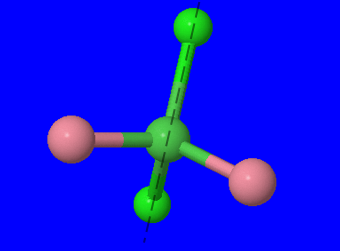(Fig 2.) We can rotate the structure and find that there is indeed a plane of symmetry through the two chloro ligands and central atom and between the carbonyl ligands.

Since there is a plane of symmetry, we can conclude that there are no optical isomers.

Overall, there are no isomers that exist for this compound.

2. trigonal byprimidal $$\mathrm{[Mn(CO)_4(NO)]}$$(Fig 3.) The central purple atom is manganese, the carbonyl ligands are the pink atoms, and the nitrosyl ligand is the fuschia atom.

There are no ions, other coordination complex, and ambidentate ligands. Therefore, no structural isomers exist for this structure.

Geometric isomers do not exist for this compound because there is only one nitrosyl ligand.(Fig 4.) Dashed line bisects molecule and shows plane of symmetry. The molecule is rotated in this image.

In the image above, after the structure has been rotated, we can see that there is a plane of symmetry. Thus, there are no optical isomers.

No isomers (the ones mentioned above) exist for this compound.

3. $$\mathrm{[Pt(en)_{2}Cl_2]Cl_2}$$(Fig 5.) The green atoms are the chloro ligands, the the central atom is platinum, and the grey/blue atoms are ethyldiamine ligands.

Coordination isomerism cannot exist for this complex because there are no other complexes. There are no linkage isomers because there are no ambidentate ligands. Ionization isomers cannot exist in this complex either, even though there is a neutral molecule outside the coordination sphere. If we exchange $$\mathrm{Cl_2}$$ with one ethyldiamine molecule, There would be 5 ligands in the coordination sphere instead of 4. This difference in the ratio of metal atom to ligands means that an ionization isomer cannot exist.(Fig 6.) Here, one chloro ligand exchanged places with the ethyldiamine so that it can be at a 90° angle with the other chloro ligand.

The image above, shows the chloro and ethyldiamine ligands at a 90° angle with its other identical ligand. This is the cis isomer, while Fig. 5 shows the trans isomer.

Fig 5. shows that there is a plane of symmetry in the trans isomer. Therefore, that structure does not have an optical isomer. On the other hand, the cis isomer does not have a plane of symmetry and therefore has an optical isomer.

### A19.2.8

none; none; The two Cl ligands can be cis or trans. When they are cis, there will also be an optical isomer.

### Q19.2.9

Predict whether the carbonate ligand $$\ce{CO3^2-}$$ will coordinate to a metal center as a monodentate, bidentate, or tridentate ligand.

### S19.2.9$$\ce{CO3^2−}$$ can be either monodentate or bidentate, since two of its oxygen atoms have lone pairs as shown above and can form covalent bonds with a transition metal ion. In most cases carbonate is monodentate because of its trigonal planar geometry (there is 120 degrees between the oxygens so it's hard for both to bind to the same metal). However, in some cases it will bind to two different metals, making it bidentate.

### A19.2.9

CO3-2 will coordinate to a metal center as a monodentate ligand.

### Q19.2.10

Draw the geometric, linkage, and ionization isomers for [CoCl5CN][CN].

### S19.2.10

Isomers are compounds with same formula but different atom arrangement. There are two subcategories: structural isomers, which are isomers that contain the same number of atoms of each kind but differ in which atoms are bonded to one another, and stereoisomers, isomers that have the same molecular formula and ligands, but differ in the arrangement of those ligands in 3D space.

There are three subcategories under structural isomers: ionization isomers, which are isomers that are identical except for a ligand has exchanging places with an anion or neutral molecule that was originally outside the coordination complex; coordination isomers, isomers that have an interchange of some ligands from the cationic part to the anionic part; and linkage isomers, in two or more coordination compounds in which the donor atom of at least one of the ligands is different.

There are also two main kinds of stereoisomers: geometric isomers, metal complexes that differ only in which ligands are adjacent to one another (cis) or directly across from one another (trans) in the coordination sphere of the metal, and optical isomers, which occurs when the mirror image of an object is non-superimposable on the original object.

Some of the isomers look almost identical, but that is because the CN ligand can be attached by both (but not at the same time) the C or N.

### A19.2.1010.P: Problems is shared under a not declared license and was authored, remixed, and/or curated by LibreTexts.